# Shadow

A meter pole perpendicular to the ground throws a shadow of 40 cm long, the house throws a shadow 6 meters long. What is the height of the house?

Result

h2 =  15 m

#### Solution:Leave us a comment of example and its solution (i.e. if it is still somewhat unclear...):

Showing 0 comments:Be the first to comment!#### To solve this example are needed these knowledge from mathematics:

See also our trigonometric triangle calculator.

## Next similar examples:

1. Tree shadowThe shadow of the tree is 16 meters long. Shadow of two meters high tourist sign beside standing is 3.2 meters long. What height has tree (in meters)?
2. Shadow and light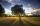Nine meters height poplar tree has a shadow 16.2 meters long. How long shadow have at the same time Joe if he is 1,4m tall?
3. Liters od milkThe cylinder-shaped container contains 80 liters of milk. Milk level is 45 cm. How much milk will in the container, if level raise to height 72 cm?
4. DonutsFind how many donuts each student will receive if you share 126 donuts in a ratio of 1:5:8
5. AnglesIn the triangle ABC, the ratio of angles is: a:b = 4: 5. The angle c is 36°. How big are the angles a, b?
6. Center traverseIt is true that the middle traverse bisects the triangle?
7. MedianThe median of the triangle LMN is away from vertex N 84 cm. Calculate the length of the median, which start at N.
8. Smallest internal angle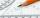Calculate what size has the smallest internal angle of the triangle if values of angles α:β:γ = 3:4:8
9. Acute angles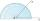Sizes of acute angles in the right-angled triangle are in the ratio 1: 3. What is size of the larger of them?
10. Type of triangle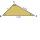How do I find the triangle type if the angle ratio is 2:3:7 ?
11. Find the 9Find the missing angle in the triangle and then name triangle. Angles are: 95, 2x+15, x+3
12. Sides od triangleSides of the triangle ABC has length 4 cm, 5 cm and 7 cm. Construct triangle A'B'C' that are similar to triangle ABC which has a circumference of 12 cm.
13. Book readIf Petra read 10 pages per day, she would read the book two days earlier than she read 6 pages a day. How many pages does a book have?
14. Angles ratioIn a triangle ABC true relationship c is less than b and b is less than a. Internal angles of the triangle are in the ratio 5:4:9. The size of the internal angle beta is:
15. Reciprocal equationDetermine the root of the equation: 9/x-7/x=1
16. SlopeFind the slope of the line: x=t and y=1+t.
17. Cows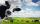4 cows spent 16 bags of hay in 5 days. How many bags of hay sacks is needed for 5 cows for seven days?